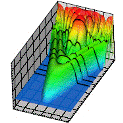## Research Papers in Physics and Astronomy## Anthony F. Starace Publications

5-1-1998

#### Comments

Published by American Physical Society. Phys. Rev. A 57, 3233 (1998). http://pra.aps.org. Copyright © 1998 American Physical Society. Permission to use.

#### Abstract

Standard representations of finite rotation matrices (FRM) are defined by expressions that are wedded to two particular coordinate frames such as, e.g., are involved in the definition of Euler angles. We present here representations for the FRM in invariant tensor forms comprised of vectors defined in a space-fixed coordinate frame K. Three explicit expressions for a FRM are presented: First, in terms of tensor products of the spherical or Cartesian basis vectors of frame K, second in a differential form containing the tensor products of gradient operators, and, third, as the superposition of so-called “minimal” bipolar harmonics depending on any pair of unit vectors a, b connected with a frame K. Based on these results for the FRM, the transformation rule for an irreducible tensor set under space rotations may be written in terms of bipolar harmonics. Our results are especially useful for analyzing angular distributions in atomic processes involving a precise accounting of all effects of photon and target polarizations. Four examples are considered as illustrations of the techniques presented. First, an invariant representation of the photon polarization tensor is found in terms of linear and circular polarization degrees of the photon beam. Second, an invariant decomposition of tripolar harmonics of second rank in terms of very simple, rank 2 tensors is presented. Third, a convenient parametrization is proposed for the polarization state multipoles of a polarized atomic target. Fourth, a simple invariant formula is derived for the angular distribution of polarized photons resulting from electric dipole photon emission by an arbitrary polarized atom.

COinS